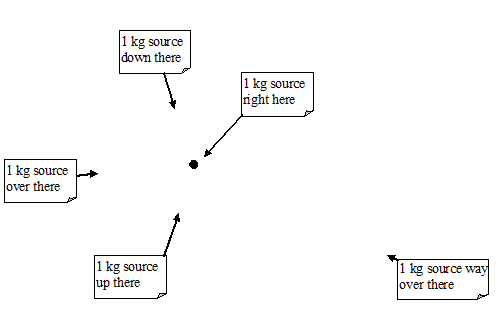$$\require{cancel}$$

# 1.1: Concepts and Principles

•• Contributed by Paul D'Alessandris
• Professor (Engineering Science and Physics) at Monroe Community College

# Electric Charge, Electric Field and a Goofy Analogy

We all know that electrons and protons have electric charge. But what is electric charge and what does it mean for a particle, like a neutron, to not have electric charge? On one level, the answer is that electric charge is the ablility to create and interact with an electric field. Of course, this begs the question, what is an electric field? To try to answer this question, let's look at mass and the gravitational field.

In Newton's theory of gravitational, every object that has mass creates a gravitational field. The object with mass is termed the source of the gravitational field. The source's gravitational field, which fills all of space, encodes information as to the location and mass of the source into space itself. It's almost as if an infinite number of little business cards have been printed and distributed throughtout space with detailed information concerning the source's characteristics. The gravitational field can be thought of as a huge number of business cards embedded into the fabric of space.(No, I am not joking, it looks like this.)Typically when we draw the gravitational field we translate the message on the business cards into a mathematically equivalent message. Here's a picture of the gravitational field near the surface of the earth.

pic

The relationship that allows you to "translate the business cards" is Newton's expression for the gravitational field surrounding a mass,

pic

where

• G is the gravitational constant, equal to 6.67 x 10-11 N m2/kg2.
• m is the source mass, the mass that creates the gravitational field.
• r is the distance between the source mass and the location of the business card.
• (r hat) is the unit vector that points from the source mass to the business card. Notice that the direction of the gravitational field, g, is opposite to this direction.

Every mass in the universe has a gravitational field described by this formula surrounding it. Additionally, every mass in the universe has the ability to "read the business cards" of every other mass. This transfer of information from one mass to another is the gravitational force that attracts masses together. (Once one mass reads another masses' business card, the first mass feels a strange urge to go visit the second mass. The more enticing the business card (the larger the value of g) the stronger the urge.)

Let's drop the business card analogy and return to electric charges and electric fields. Basically, every object that has electric charge surrounds itself with an electric field given by a formula incredibly similar to the one for the gravitational field. How incredibly similar? Below is the formula for the electric field at a particular point in space, from a single source charge:

pic

where

• k is the electrostatic constant, equal to 9.00 x 109 N m2/C2.
• q is the source charge, the electric charge that creates the electric field. Its value can be positive or negative, and is measured in coulombs (C).
• r is the distance between the source charge and the point of interest.
• (r hat) is the unit vector that points from the source charge to the point of interest.

Every charge object is surrounded by a field given by this relationship. Every other charged object in the universe can "read" this field and will respond to its information by feeling an electric force. Object without electric charge neither create nor interact with electric fields (they can't read the business cards).

In this chapter you'll learn how to calculate the electric field produced by charge objects. In the next chapter, you'll learn how the electric field can be "sensed" by other electric charges resulting in the electric force.

 Actually, neutrons are constructed from smaller particles called quarks that do have electric charge. Neutrons are neutral because the sum of the quark charges inside of them is zero. The distinction between an object having a net charge of zero and an object having no charge whatsoever is important when considering polarization.